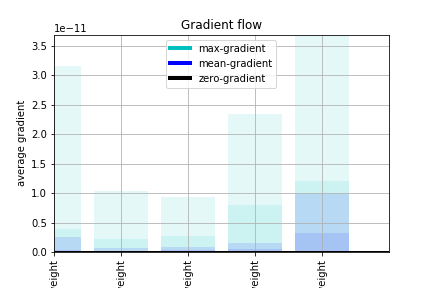I’ve been working on an unbalanced binary classification problem, where true to false ratio is 9:1, and my input is 20 dim tabular data. In order to handle this imbalanced dataset, I decided to use Focal loss. My implementation of this metric is a PyTorch adaptation of the Tensorflow one.

I made a couple of tests and the outcome of my focal loss implementation is the same as the one produced by the TensorFlow one.

``````class FocalLoss(nn.Module):
"""
Weighs the contribution of each sample to the loss based in the classification error.
:gamma: Focusing parameter. gamma=0 is equivalent to BCE_loss
"""
def __init__(self, gamma, eps=1e-6):
super(FocalLoss, self).__init__()
self.gamma = gamma

def forward(self, y_pred, y_true):
y_true = y_true.float()
pred_prob = torch.sigmoid(y_pred)
ce = nn.BCELoss(reduce=False)(pred_prob,y_true)

p_t = (y_true*pred_prob)+((1-y_true)*(1-pred_prob))
modulator = 1.0
if self.gamma:
modulator = torch.pow((1.0-p_t),torch.tensor(self.gamma).to(device) )